## What are similar triangles?

Similar triangles are triangles in which their corresponding angles are congruent and their corresponding sides are proportional. They are the same shape but not basically the same size.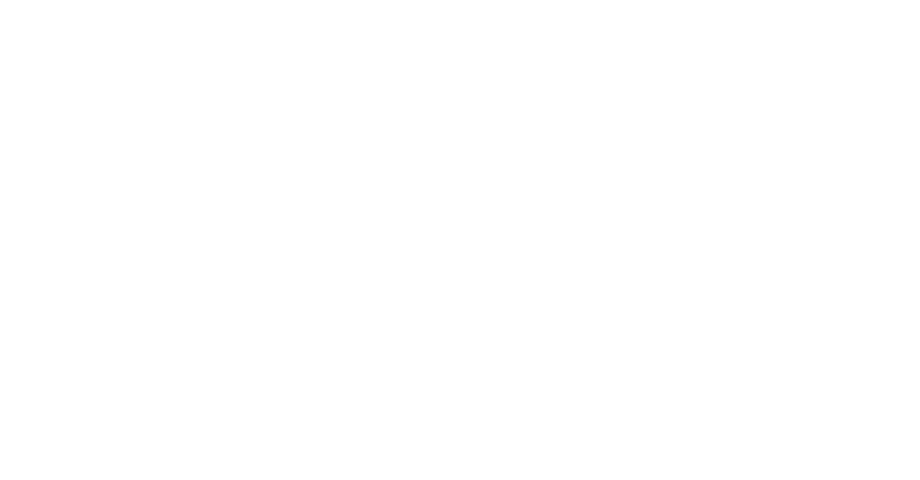### Three rules to prove if two triangles are similar

1. AA – the Angle-Angle rule states that if two corresponding angles of our two triangles are equal than these two triangles are similar.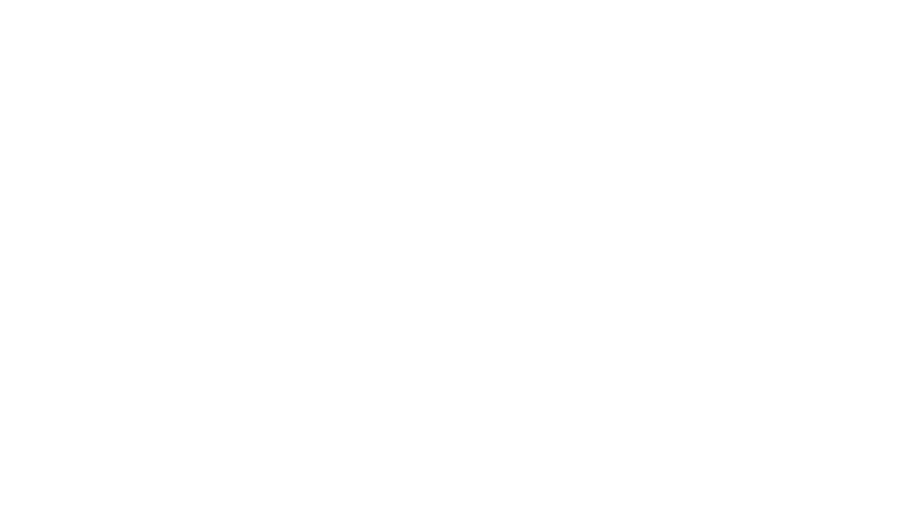2. SSS – the Side-Side-Side rule states that if in two triangles the three corresponding sides are proportional with each other than these two triangles are similar.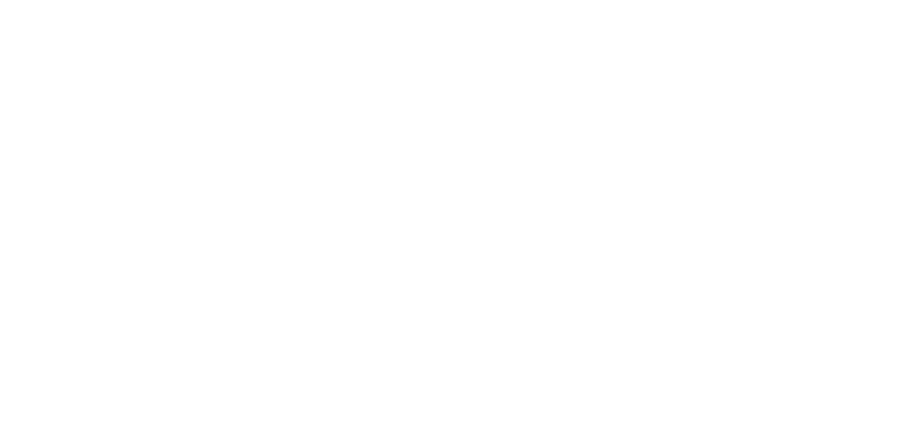3. SAS – the SideAngleSide rule states that if in two triangles the two corresponding sides are proportional and the corresponding angle between them is equal than these two triangles are similar.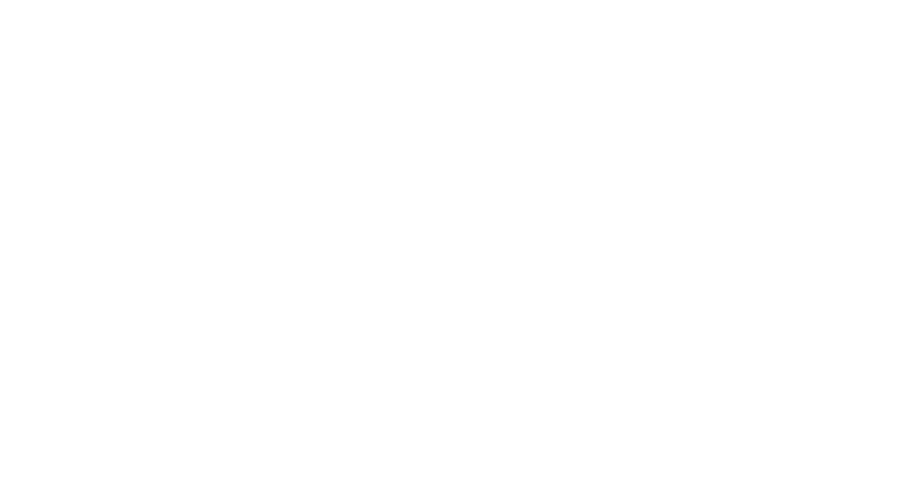## Example 1: You are given two triangles. Find the length of x.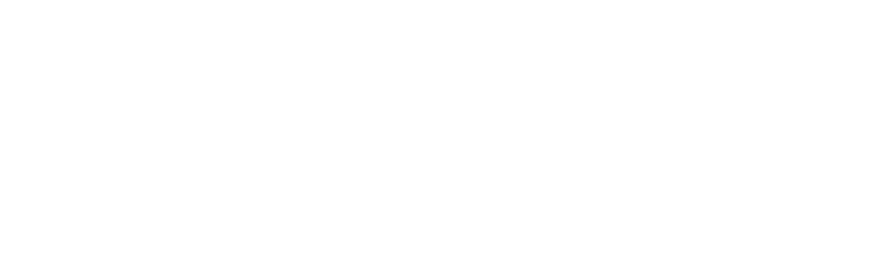Step 1: This two triangles are similar based on the AA rule the two triangles are congruent.

Step 2: Since this two triangles are congruent then all the sides are proportional ,the ratio of the corresponding length sides is the same:

$\displaystyle \frac{10}{5}=\frac{8}{x}$

Step 3: Find the x by using basic algebra:

$\displaystyle 10x=8\cdot 5$

x = 4

Example 2: Explain why the two triangles shown on the diagram are similar and work out x and y.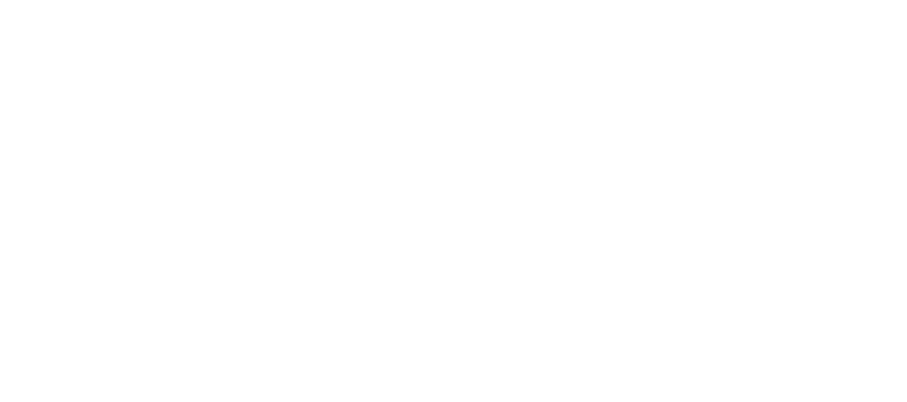Solution: $\displaystyle \angle$ACB = 180°- 35° – 100° = 45°.

$\displaystyle \angle$FED = 180° – 45° – 35° – 100° = 35°.

So, both triangles have exactly the same three angles and are therefore, similar.

Since the triangles are similar:$\displaystyle \frac{DE}{AB}=\frac{EF}{BC}=\frac{DF}{AC}$

So$\displaystyle \frac{y}{7}=\frac{12}{4}=3m$

y = 3 x 7 = 21 and $\displaystyle \frac{6}{x}=\frac{12}{4}=3$

$\displaystyle x=\frac{6}{3}=2m$

Example 3: In the triangle below B’C’ is parallel to BC, find the length x of B’B and y of AC’.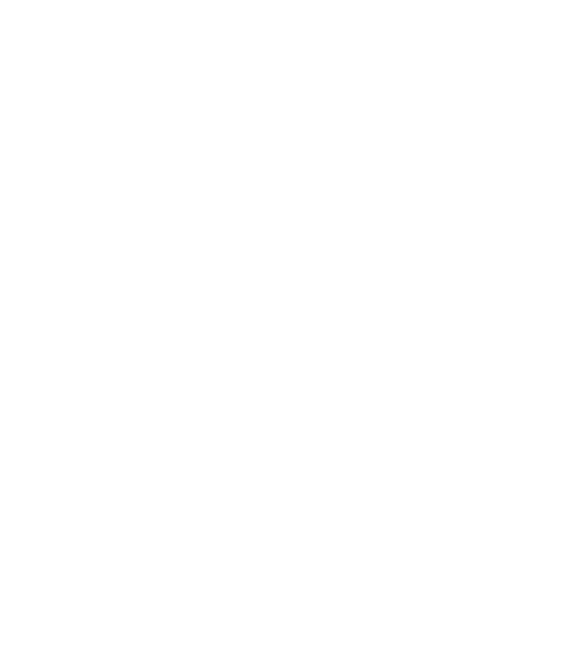BA is a line that intersects the two parallel lines BC and B’C’, so the corresponding angles are congruent.

$\displaystyle \widehat{A{B}'{C}’}=\widehat{ABC}$

AC is a line that intersects the two parallel lines BC and B’C’, so the corresponding angles are congruent.

$\displaystyle \widehat{ACB}=\widehat{AC’B’}$

The triangles ABC and AB’C’ are similar since they have two congruent corresponding angles. Since they are similar the lengths of their sides are proportional.

Our two Triangles: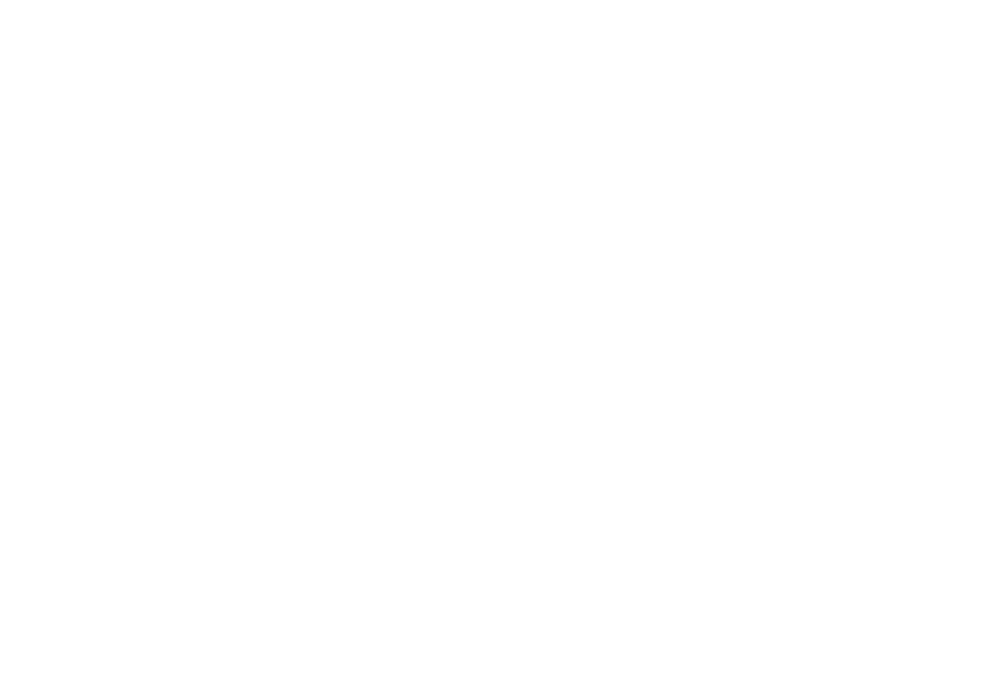Using the fact that the side lengths are proportional in two similar triangles we write  that will help us to find x and y.

$\displaystyle \frac{7+x}{7}=\frac{10}{5}=\frac{y+4}{y}$

Write the equation to find the x.

$\displaystyle \frac{7+x}{7}=\frac{10}{5}$

$\displaystyle \frac{7+x}{7}=2$

7 + x = 14

x = 7

Write the equation to find the y.

$\displaystyle \frac{y+4}{y}=\frac{10}{5}$

$\displaystyle \frac{y+4}{y}=2$

x + 4 = 2y

y = 4

So, we found that x = 7 and y = 4.